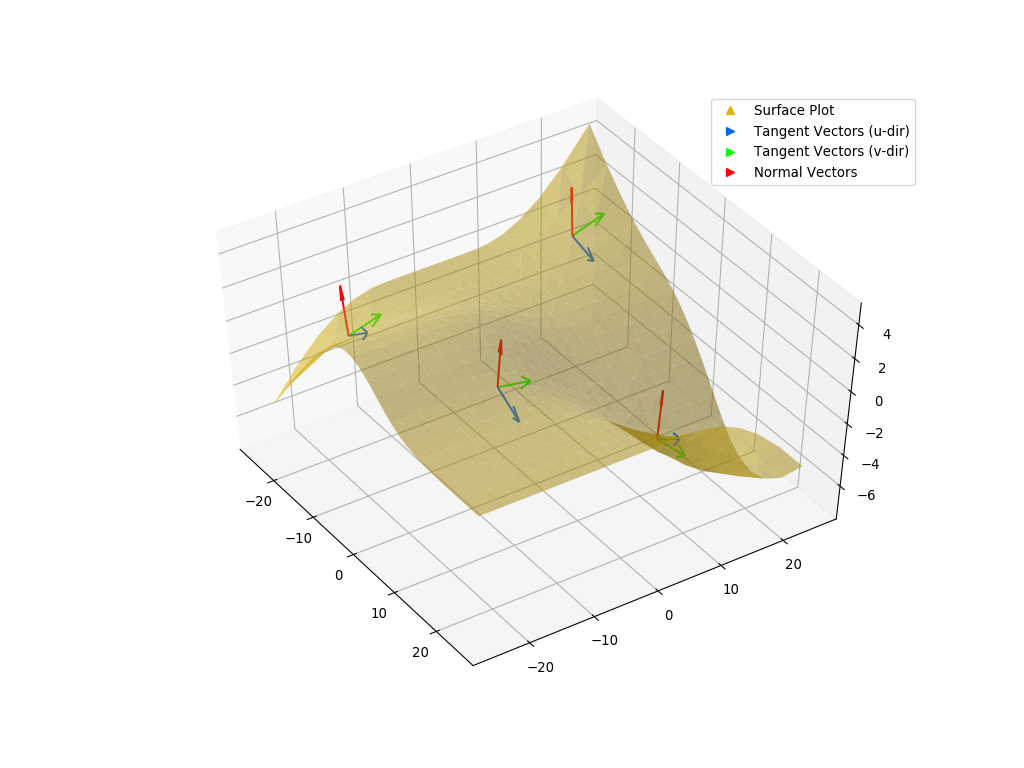# Visualization¶

NURBS-Python comes with the following visualization modules for direct plotting evaluated curves and surfaces:

Examples repository contains over 40 examples on how to use the visualization components in various ways. Please see Visualization Modules Documentation for more details.

## Examples¶

The following figures illustrate some example NURBS and B-spline shapes that can be generated and directly visualized via NURBS-Python.

### Curves¶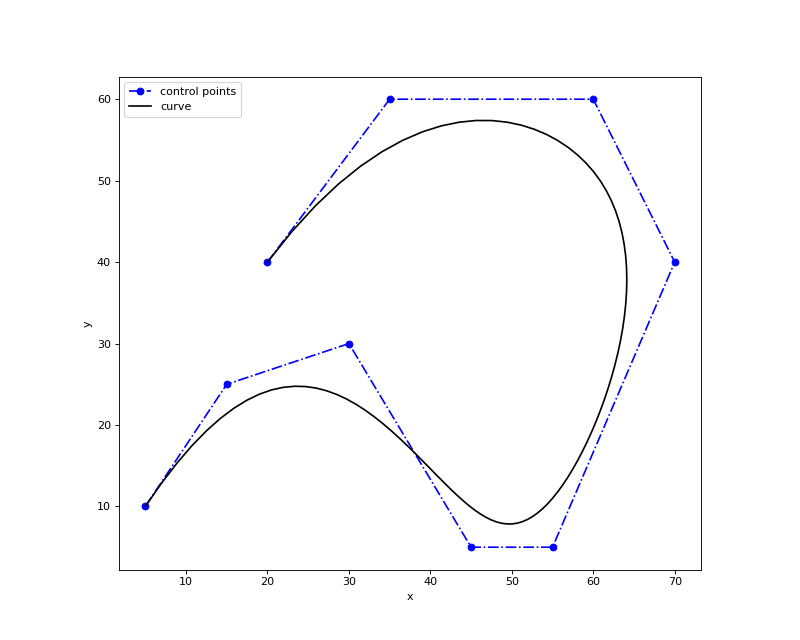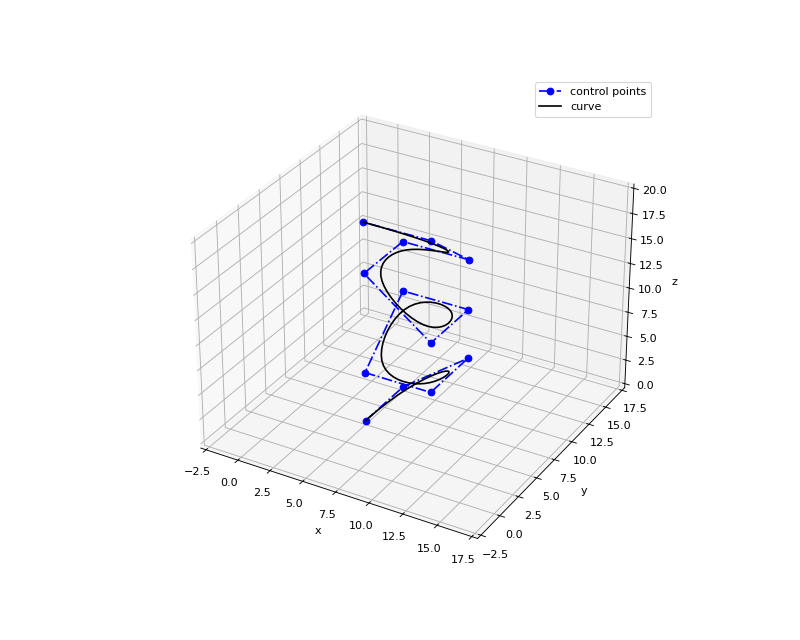### Surfaces¶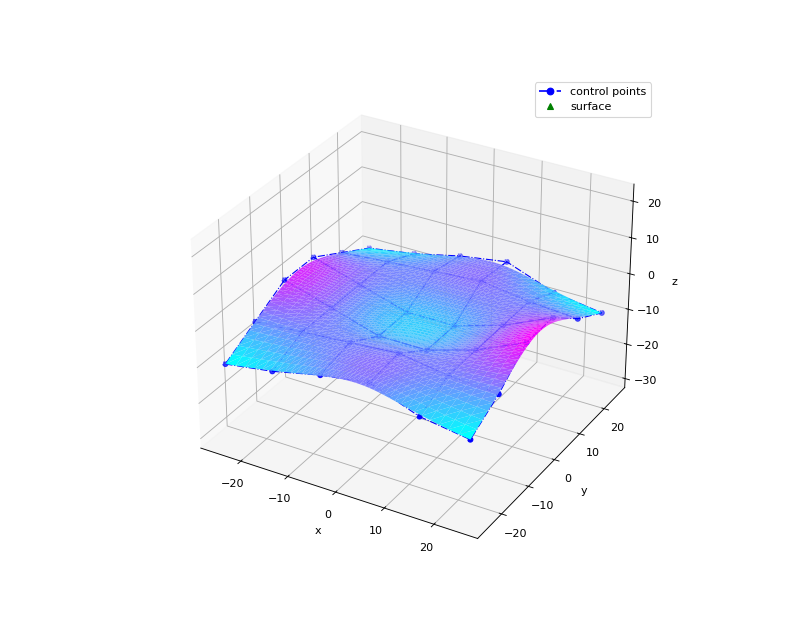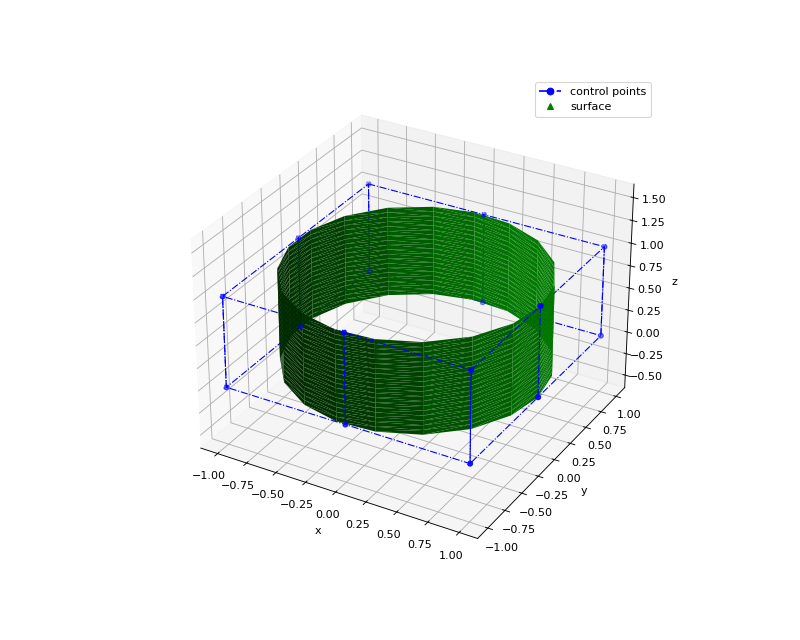### Volumes¶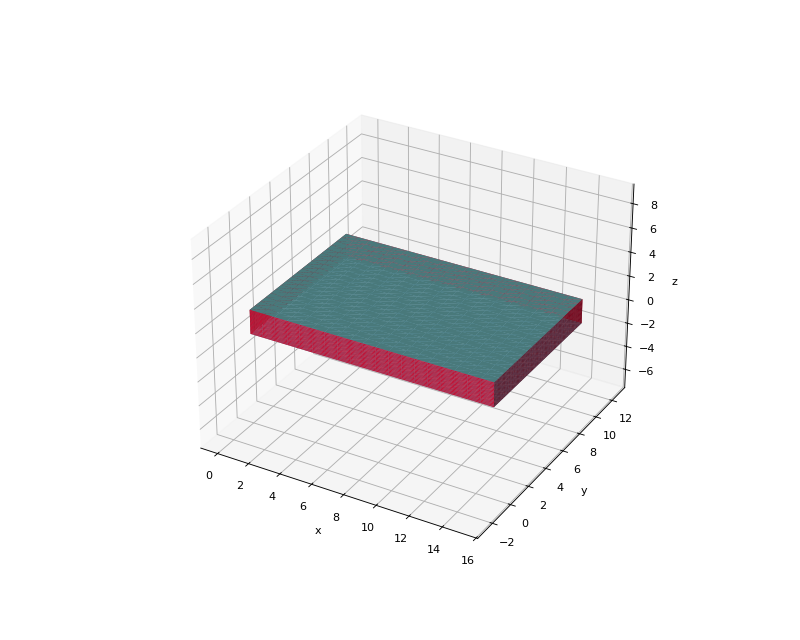The following example scripts can be found in Examples repository under the `visualization` directory.

#### mpl_curve2d_tangents.py¶

This example illustrates a more advanced visualization option for plotting the 2D curve tangents alongside with the control points grid and the evaluated curve.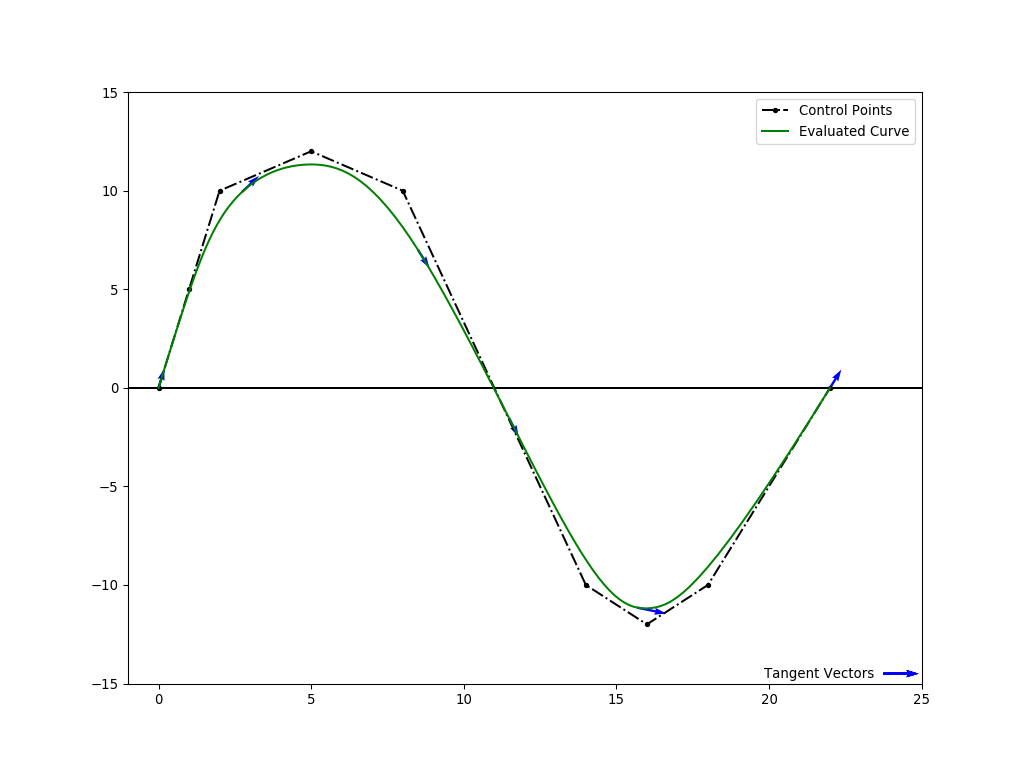#### mpl_curve3d_tangents.py¶

This example illustrates a more advanced visualization option for plotting the 3D curve tangents alongside with the control points grid and the evaluated curve.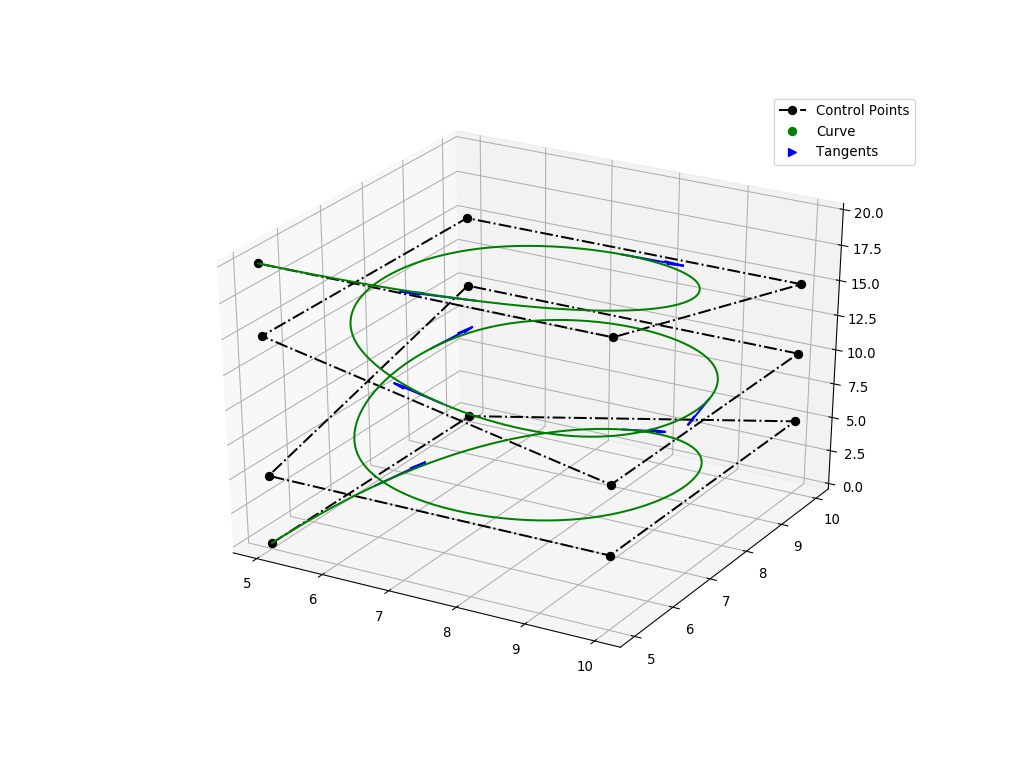#### mpl_curve3d_vectors.py¶

This example illustrates a visualization option for plotting the 3D curve tangent, normal and binormal vectors alongside with the control points grid and the evaluated curve.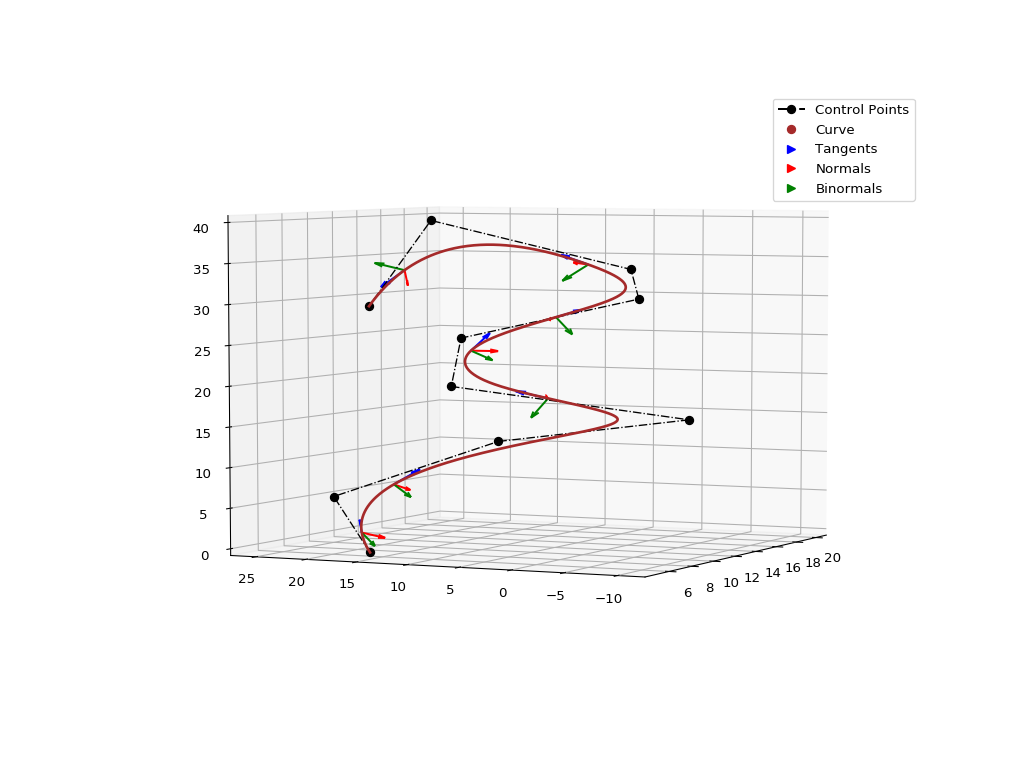#### mpl_trisurf_vectors.py¶

The following figure illustrates tangent and normal vectors on `ex_surface02.py` example.